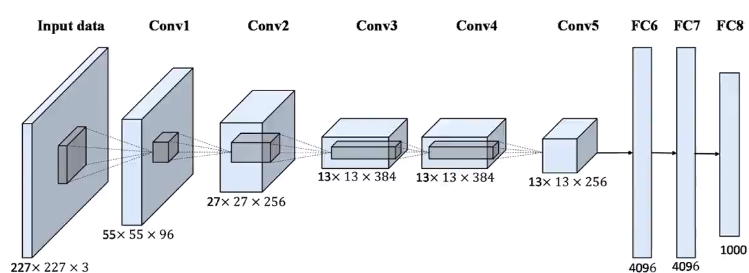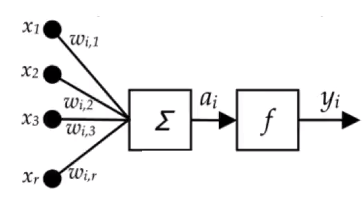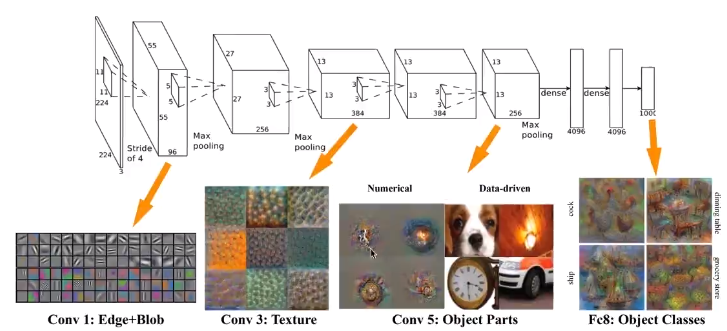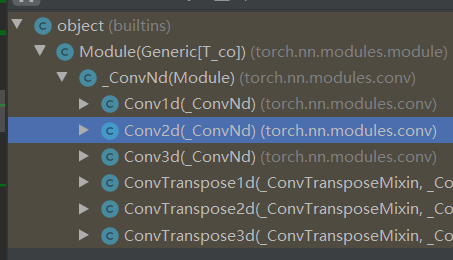2020/02/23 22:10

# 神经网络概述x表示输入，x1，x2，...， xr

w表示权值，wi1，wi2，...，wir。其中下标中第一个值i对应于整个神经元的最终输出yi的下标i；第二个下标1....r表示输入x的下标值

α是求和后得到的值

y表示神经元的输出w的值再最开始都是随机的，或者可以定义成0或者1，注意不能全0。

（本文出自oschina博主happyBKs的博文：https://my.oschina.net/happyBKs/blog/3171003）

Pytorch所有神经网络的核心，这个包完成了所有梯度计算、反向传递的过程。

import torch

# 创建一个2d tensor，2乘2的矩阵，元素全为1，类型<class 'torch.Tensor'>
print(x)

tensor([[1., 1.],
[1., 1.]], requires_grad=True)

y = x + 2
z = y * y
out = z.mean()
print("y:", y)
print("z:", z)
print("out:", out)

# 计算梯度、反向传播
out.backward()

# 打印梯度值
print("grad:", x.grad)

grad: tensor([[1.5000, 1.5000],
[1.5000, 1.5000]])

# 如果忽略梯度，看看结果
k = x + 1
print("k:", k)

# 神经网络的训练过程import torch
import torch.nn as nn
import torch.nn.functional as F

class HappyBKsNet(nn.Module):

def __init__(self):
super(HappyBKsNet, self).__init__()
# 定义神经网络基本结构
# 输入数据的格式 为 1x32x32，通道数x图像像素行数x图像像素列数。例如，rgb图片通道是为3，所以输入数据应该是3x32x32
# 第一层（卷积层）
# Conv2d为2维卷积层的类，三个参数分别为（输入通道数量，输出通道数量， 卷积大小3x3）
self.conv1 = nn.Conv2d(1, 6, 3)
# 第二层（卷积层）
# 第二层卷积层的输入通道是第一层输出的通道，所以数量都是6；第二层卷积层输出通道数是16；并且本层还是用3x3的卷积
self.conv2 = nn.Conv2d(6, 16, 3)
# 第三层（全连接层）
# 全连接层在pytorch的nn中又叫线性层，用Linear类创建。其有2个参数，（输入通道数量，输出通道数量)
# 注意，全连接层的输入数量不单单是上一层卷积层的输出16，而是16 * 28 * 28 = 12544。这里的28是我们神经网络的输入32x32，经过两次卷积之后，每次减2，得到32 - 2 -2 = 28
self.fc1 = nn.Linear(16 * 28 * 28, 512)
# 第四层（全连接层）
# 上一层已经是全连接层了，所以上一层输出的就是一个1*n的向量。所以按照上一层全连接层输出的向量尺寸输入本层全连接层即可。
# 输入维度512， 输出维度64
self.fc2 = nn.Linear(512, 64)
# 第五层（全连接层）
# 输入维度64， 输出维度2
self.fc3 = nn.Linear(64, 2)

def forward(self, *input, **kwargs):
# 定义数据流向
# 输入数据为x
x = input
# 输入数据输入第一层conv1
x = self.conv1(x)
# 第一层之后，我们要做一个激活：把x值变换到一个固定的范围内
# 激活变换到的数据范围，可以是0~1， -1~1， 0~+∞，对应于不同的激活函数
# 这里我们使用relu
x = F.relu(x)
# 将第一层的输出传入第二层中，也同样再做一个激活
x = self.conv2(x)
x = F.relu(x)

# 从卷积层到全连接层，数据的形状是要发生变化的。和上面定义网络结构时，定义输出输入参数一样，这里也需要对输入数据做一个形状变换。
# 我们需要把上一层数据的输出形状变一下，
x = x.view(-1, 16 * 28 * 28)
# 将变换形状后的输入传入下一层全连接层中。
x = self.fc1(x)
x = F.relu(x)

x = self.fc2(x)
x = F.relu(x)

x = self.fc3(x)

return x

if __name__ == "__main__":
happyBKsNet= HappyBKsNet()
print(happyBKsNet)Net(
(conv1): Conv2d(1, 6, kernel_size=(3, 3), stride=(1, 1))
(conv2): Conv2d(6, 16, kernel_size=(3, 3), stride=(1, 1))
(fc1): Linear(in_features=12544, out_features=512, bias=True)
(fc2): Linear(in_features=512, out_features=64, bias=True)
(fc3): Linear(in_features=64, out_features=2, bias=True)
)### 作者的其它热门文章

0
0 收藏

0 评论
0 收藏
0